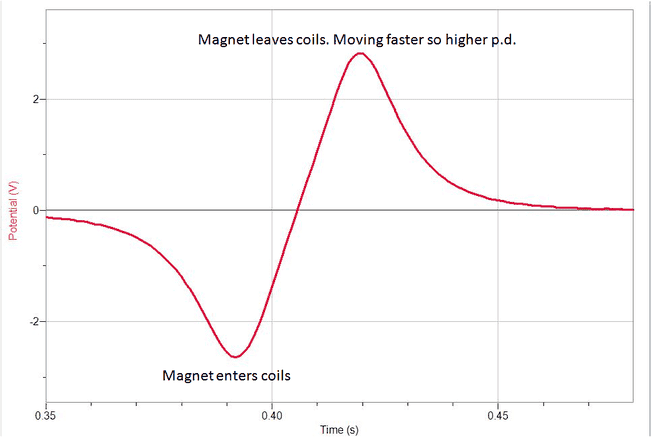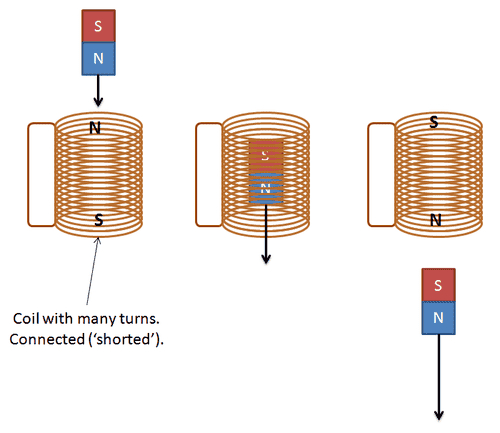# Magnetism in coils

Hannah7hThis is a graph for a magnet falling through a coil, it shows the p.d induced in the coil (the induced emf) when the magnet enters and then shows the induced emf in the coil when the magnet leaves.
My question is why does the p.d appear to change direction? (i.e why the positive and negative values?)

## Answers and Replies

Staff Emeritus
When the magnet is entering the coil, what is happening to the magnetic flux through the coil? What about when it is leaving the coil? What do you know about induction and its relation to the change in the magnetic flux?

Hannah7h
When the magnet is entering the coil, what is happening to the magnetic flux through the coil? What about when it is leaving the coil? What do you know about induction and its relation to the change in the magnetic flux?

I know that an emf will be induced in the coil when there is a change in magentic flux, and that if the coil is connected in a closed circuit, a current will also be induced in the coil. I also know that the direction of this current is in such a direction to create a magnetic field which opposes the change that caused the induced current in the first place (Lenz's law). So I guess when the magnet enters the coil, there is a change in magnetic flux which induces an emf in the coil in one direction, and then when the magnet leaves the coil there is another change in flux and it induces an emf in the coil in the opposite direction, hence the positive and negative values? Is this the reason for the +ve and -ve values?

2) Also if the magnet entered the coil with its North pole, there would be a change in flux in the coil, an emf would be induced and then a current would be induced in the coil, which would generate a North magnetic field in the coil, to oppose the motion of the magnet. But I was wondering why, when the magnet leaves the coil, is another North magnetic field generated in the bottom of the coil, i.e. why do the poles on the coil switch? I have attached a picture below to try and show what I'm talking aboutnasu
In the second case, the magnet leaves the coil. How would you have the poles on the coil in order to oppose the magnet leaving the coil? Would you have the coil attract or repel the magnet, in the third drawing?

Hannah7h
In the second case, the magnet leaves the coil. How would you have the poles on the coil in order to oppose the magnet leaving the coil? Would you have the coil attract or repel the magnet, in the third drawing?
In the second case, the magnet leaves the coil. How would you have the poles on the coil in order to oppose the magnet leaving the coil? Would you have the coil attract or repel the magnet, in the third drawing?

You would have the coil attracting the magnet, therefore the the pole on the coil would be a north pole to attract the south pole of the magnet to oppose its motion. Ah yes I get it now, thank you